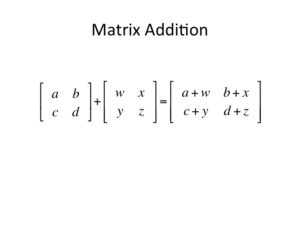# Java Program to Add Two Matrices – 4 Ways | Programs

Java program to add two matrices – The following Java Code will let you know how to perform two matrix addition using Java. Soon we will add compiler to execute the Program below each method. We have written the Program in 3 Possible ways. Here it is,

• Using For Loop
• Using While
• Using Do-While

Addition of two matrices can be carried if and only if both the matrices are in the same order. In matrix addition, each term of one matrix is added to the other matrix’s term, at the same location, i.e. the term at first row first column of Matrix 1 will be added to the term at first row first column of Matrix 2 and so on. A pictorial example is given below.## Addition Of Two Matrices – Using For Loop

1) If both matrices are of the same size then only we can add the matrices.

2) Use the double dimensional array to store the matrix elements.

3) Read row number,column number and initialize the  double dimensional arrays mat1[][],mat2[][],res[][] with same row number,column number.

4) Store the first matrix elements into the two-dimensional array mat1[][] using two for loops. i indicates row number, j indicates column index.Similarly matrix 2 elements in to mat2[][].

5) Add the two matrices using for loop

• for i=0 to i<row
• for j=0 to j<col
• mat1[i][j] + mat2[i][j] and store it in to the matrix res at res[i][j] .

Output:

### Using While Loop

1) For insert values in the matrices mat1, mat2 using loops –

• While loop iterates until i <row
• while iterates until  j<column
• insert the value at  mat1[i][j] and increases the j value.
• increase the i value.

• while loop iterates until i<row
• while loop iterates until j<column
• add  mat1[i][j] + mat2[i][j] and insert the result at res[i][j].
• increase the j value.
• increase the i value.

3) Print res[i][j] using two for loops then we will get the addition of two matrices.

Output:

##### Using Do-While Loop

1) For Addition of two matrices

• i=0
• do loop
• j=0
• do loop
• mat1[i][j] + mat2[i][j] and insert the result in to res[i][j].
• Increase the j value. checks the condition j<col.
• Increase the i value. Then checks the condition j<row.

2) Print res[][] then we will get the addition of two matrices.

Output:

More Java Programs:

x

## Java Program To Subtract Two Matrices – 3 Ways

Java program for matrix subtraction. The following Java code to subtract two matrices has been ...